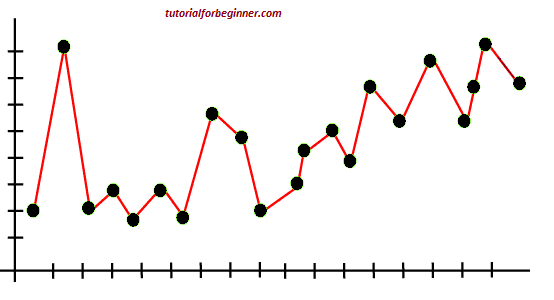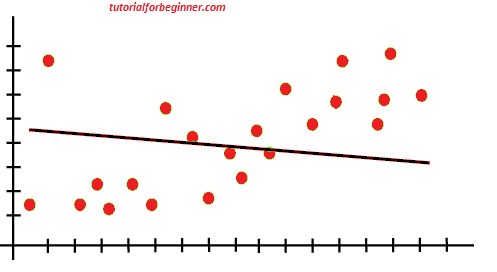# Overfitting and Underfitting in Machine Learning

In this page, we will learn Overfitting and Underfitting in Machine Learning, Overfitting, How to avoid the Overfitting in Model, Underfitting, How to avoid underfitting, Goodness of Fit.

The two most common difficulties in machine learning are overfitting and underfitting, both of which affect the performance of machine learning models.

Each machine learning model's key goal is to generalize well. The capacity of an ML model to offer an acceptable output by adapting the provided set of unknown input is referred to as generalization. It indicates that after training on the dataset, it can give accurate and dependable results. As a result, the two words underfitting and overfitting must be examined for the model's performance and whether it is generalizing well or not.

Before we get into overfitting and underfitting, let's define a few terms that will help us better comprehend this topic:

• Signal: The genuine underlying pattern of the data that aids the machine learning model in learning from the data is referred to as the signal.
• Noise: It is unimportant and irrelevant input that degrades the model's performance.
• Bias: It is a prediction inaccuracy incorporated into the model as a result of oversimplifying machine learning methods. Or it could be the disparity between projected and actual numbers.
• Variance: It occurs when a machine learning model performs well on the training dataset but not on the test dataset.

## Overfitting

When our machine learning model tries to cover all of the data points in a dataset, or more than the required data points, overfitting occurs. As a result, the model begins to cache noise and erroneous values from the dataset, all of which reduces the model's efficiency and accuracy. Low bias and large variance characterize the overfitted model.

As we supply more training to our model, the odds of overfitting rise. It indicates that the more we train our model, the more likely it is to become overfitted.

In supervised learning, overfitting is the most common issue.

The following graph of the linear regression output helps to understand the concept of overfitting:The model tries to cover all of the data points in the scatter plot, as seen in the graph above. It may appear to be efficient, but it is not. Because the regression model's purpose is to identify the best fit line, but we don't have one here, it will create prediction errors.

## How to avoid the Overfitting in Model

The machine learning model's performance is harmed by both overfitting and underfitting. However, because overfitting is the main reason, there are certain approaches to limit the likelihood of overfitting in our model.

• Cross-Validation
• Training with more data
• Removing features
• Early stopping the training
• Regularization
• Ensembling

## Underfitting

When our machine learning model is unable to capture the underlying trend of the data, we call this underfitting. To prevent the model from overfitting, the feeding of training data can be halted at an early stage, otherwise the model may not learn enough from the training data. As a result, it's possible that it won't be able to discover the best fit for the data's prevailing trend. Underfitting occurs when the model is unable to learn enough from the training data, resulting in lower accuracy and incorrect predictions.

A model that is underfitted has a high bias and a low variance.

Example: We can understand the underfitting using below output of the linear regression model:The model, as shown in the figure above, is unable to capture the data points in the plot.

## How to avoid underfitting:

• By increasing the model's training time.
• By expanding the number of features available.

## Goodness of Fit

The term "goodness of fit" is derived from statistics, and it is the purpose of machine learning models to accomplish it. It defines how closely the result or anticipated values match the true values of the dataset in statistics modeling.

The model with a good fit is halfway between underfitted and overfitted, and it gives predictions with zero errors in theory, but it's difficult to obtain in practice.

When we train our model over a period of time, the mistakes in the training data decrease, and the same is true for test data. However, if we train the model for a long time, the model's performance may suffer from overfitting, as the model learns the dataset's noise as well. The test dataset's mistakes begin to rise, thus the point right before the errors begin to rise is the good point, and we can stop here to achieve a decent model.

The resampling strategy to measure model accuracy and the validation dataset are two alternative methods for obtaining a good point for our model.